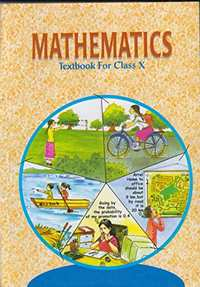Share
Notifications

View all notifications
Books Shortlist
Your shortlist is empty

# NCERT solutions for Class 10 Mathematics chapter 11 - Constructions

## Mathematics Textbook for Class 10

Login
Create free account

Forgot password?

#### NCERT Mathematics Class 10## Chapter 11: Constructions

Ex. 11.10Ex. 11.20

#### Chapter 11: Constructions Exercise 11.10 solutions [Pages 219 - 220]

Ex. 11.10 | Q 1 | Page 219

Draw a line segment of length 7.6 cm and divide it in the ratio 5:8. Measure the two parts. Give the justification of the construction.

Ex. 11.10 | Q 2 | Page 220

Construct a triangle of sides 4 cm, 5cm and 6cm and then a triangle similar to it whose sides are 2/3 of the corresponding sides of the first triangle. Give the justification of the construction.

Ex. 11.10 | Q 3 | Page 220

Construct a triangle with sides 5 cm, 6 cm and 7 cm and then another triangle whose sides are 7/5 of the corresponding sides of the first triangle. Give the justification of the construction.

Ex. 11.10 | Q 4 | Page 220

Construct an isosceles triangle whose base is 8 cm and altitude 4 cm and then another triangle whose side are 1 1/2 times the corresponding sides of the isosceles triangle.

Give the justification of the construction

Ex. 11.10 | Q 5 | Page 220

Draw a triangle ABC with side BC = 7 cm, ∠B = 45°, ∠A = 105°. Then, construct a triangle whose sides are 4/3 times the corresponding side of ΔABC. Give the justification of the construction.

Ex. 11.10 | Q 6 | Page 220

Draw a triangle ABC with BC = 7 cm, ∠B = 45° and ∠A = 105°. Then construct a triangle whose sides are4/5 times the corresponding sides of ΔABC.

Ex. 11.10 | Q 7 | Page 220

Draw a right triangle in which the sides (other than hypotenuse) are of lengths 4 cm and 3 cm. the construct another triangle whose sides are 5/3 times the corresponding sides of the given triangle. Give the justification of the construction.

#### Chapter 11: Constructions Exercise 11.20 solutions [Pages 221 - 222]

Ex. 11.20 | Q 1 | Page 221

Draw a circle of radius 6 cm. From a point 10 cm away from its centre, construct the pair of tangents to the circle and measure their lengths. Give the justification of the construction.

Ex. 11.20 | Q 2 | Page 221

Construct a tangent to a circle of radius 4 cm from a point on the concentric circle of radius 6 cm and measure its length. Also verify the measurement by actual calculation.

Ex. 11.20 | Q 3 | Page 221

Draw a circle of radius 3 cm. Take two points P and Q on one of its extended diameter each at a distance of 7 cm from its centre. Draw tangents to the circle from these two points P and Q. Give the justification of the construction.

Ex. 11.20 | Q 4 | Page 221

Draw a pair of tangents to a circle of radius 5 cm which are inclined to each other an angle of 60º.

Ex. 11.20 | Q 5 | Page 221

Draw a line segment AB of length 8 cm. Taking A as centre, draw a circle of radius 4 cm and taking B as centre, draw another circle of radius 3 cm. Construct tangents to each circle from the centre of the other circle.

Ex. 11.20 | Q 6 | Page 222

Draw a right triangle ABC in which AB = 6 cm, BC = 8 cm and ∠B = 90°. Draw BD perpendicular from B on AC and draw a circle passing through the points B, C and D. Construct tangents from A to this circle.

Ex. 11.20 | Q 7 | Page 222

Draw a circle with the help of a bangle. Take a point outside the circle. Construct the pair of tangents from this point to the circles. Give the justification of the construction.

## Chapter 11: Constructions

Ex. 11.10Ex. 11.20

#### NCERT Mathematics Class 10## NCERT solutions for Class 10 Mathematics chapter 11 - Constructions

NCERT solutions for Class 10 Maths chapter 11 (Constructions) include all questions with solution and detail explanation. This will clear students doubts about any question and improve application skills while preparing for board exams. The detailed, step-by-step solutions will help you understand the concepts better and clear your confusions, if any. Shaalaa.com has the CBSE Mathematics Textbook for Class 10 solutions in a manner that help students grasp basic concepts better and faster.

Further, we at Shaalaa.com are providing such solutions so that students can prepare for written exams. NCERT textbook solutions can be a core help for self-study and acts as a perfect self-help guidance for students.

Concepts covered in Class 10 Mathematics chapter 11 Constructions are Constructions Examples and Solutions, Construction of Tangents to a Circle, Division of a Line Segment.

Using NCERT Class 10 solutions Constructions exercise by students are an easy way to prepare for the exams, as they involve solutions arranged chapter-wise also page wise. The questions involved in NCERT Solutions are important questions that can be asked in the final exam. Maximum students of CBSE Class 10 prefer NCERT Textbook Solutions to score more in exam.

Get the free view of chapter 11 Constructions Class 10 extra questions for Maths and can use Shaalaa.com to keep it handy for your exam preparation

S